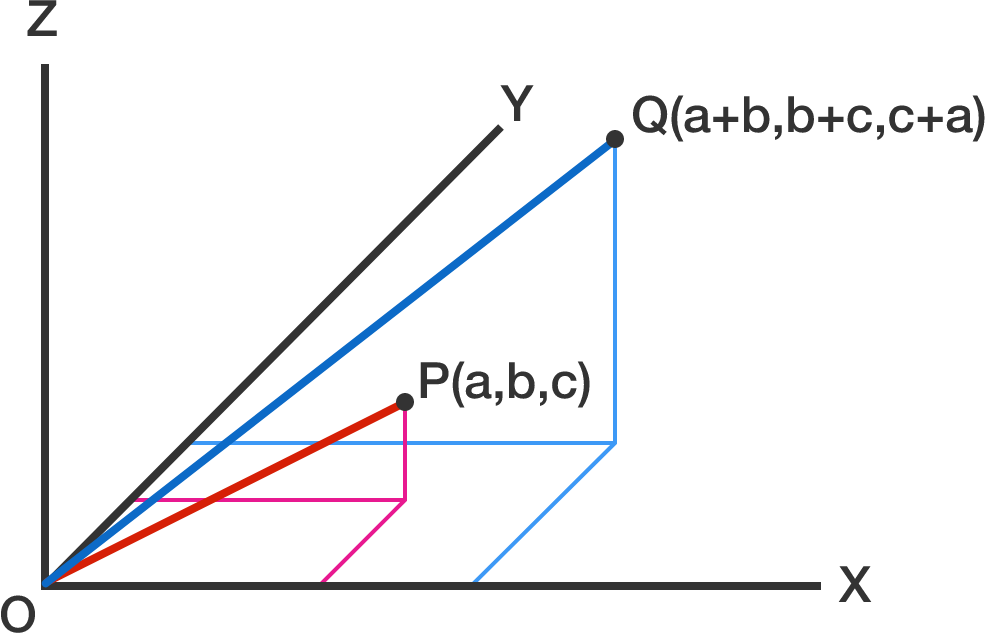# Vector RatioIn the $xyz$-plane above, let $O=(0,0,0)$ be the point of origin, $P=(a,b,c)$, and $Q=(a+b, b+c, c+a)$, where $a,b,c$ are non-zero real numbers.

What is the maximum value of the ratio $\frac{OQ}{OP}?$

×